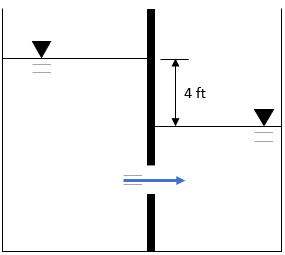## Submerged Tank

In the shown figure, two water tanks are separated by a middle divider. If a circular latch with a 10 inch diameter on the divider is opened such that water flows from the larger tank to the smaller tank, what is the common coefficient of discharge if the flow rate through the latch is 20 cubic feet per second? Assume there are no minor losses in the discharge, and steady-flow conditions.Hint
$$Q=CA\sqrt{2g(h_1-h_2)}$$$where $$Q$$ is the volumetric flow rate, $$C$$ is the coefficient of discharge, $$A$$ is the cross sectional area of flow, $$g$$ is the acceleration due to gravity, $$h_1$$ is the height of the fluid above the orifice for the taller portion, and $$h_2$$ is the height of the fluid above the orifice for the shorter portion. Hint 2 The cross sectional area for a circular latch is: $$A=\frac{\pi}{4}D^2$$$
where $$D$$ is the diameter.
Submerged orifice operating under steady flow-conditions:
$$Q=CA\sqrt{2g(h_1-h_2)}$$$where $$Q$$ is the volumetric flow rate, $$C$$ is the coefficient of discharge, $$A$$ is the cross sectional area of flow, $$g$$ is the acceleration due to gravity, $$h_1$$ is the height of the fluid above the orifice for the taller portion, and $$h_2$$ is the height of the fluid above the orifice for the shorter portion. The cross sectional area for a circular latch is: $$A=\frac{\pi}{4}D^2$$$
where $$D$$ is the diameter.
$$A=\frac{\pi}{4}(10in\cdot \frac{1ft}{12in})^2=0.545\:ft^2$$$Solving for the common coefficient of discharge: $$C=\frac{Q}{A\sqrt{2g(h_1-h_2)}}=\frac{20ft^3/s}{(0.545ft^2)\sqrt{2(32.2ft/s^2)(4ft)}}$$$
$$=\frac{20ft^3/s}{(0.545ft^2)\sqrt{257.6ft^2/s^2}}=\frac{20ft^3/s}{(0.545ft^2)(16.05ft/s)}=2.3$$\$
2.3